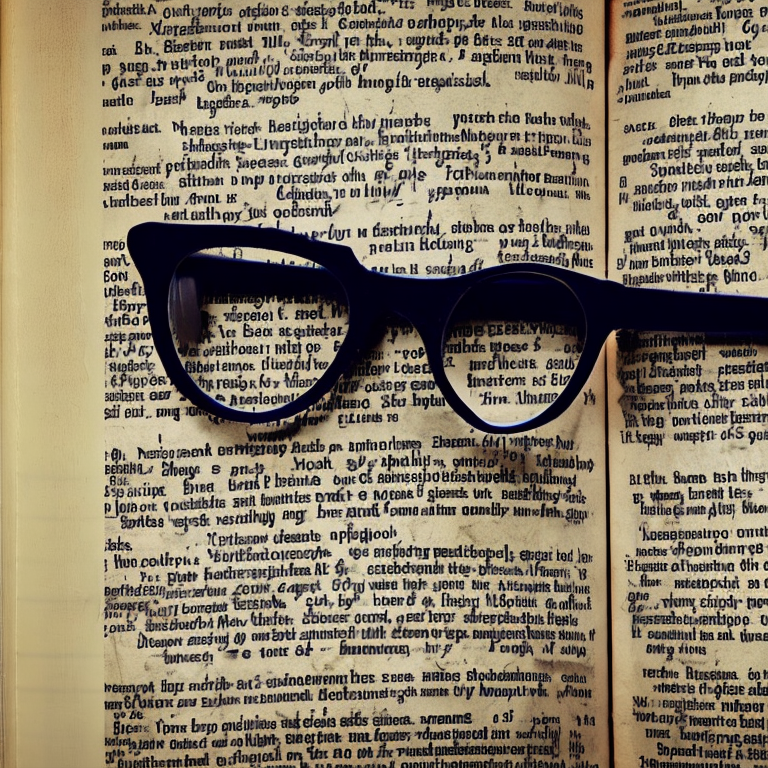# How to pre process your image for better OCR results using python

Tue, Dec 27, 2022

Optical Character Recognition (OCR) is a technology that allows computers to recognize and extract text from images and documents. In order to achieve accurate OCR results, it is important to pre-process the images in a way that maximizes the quality and legibility of the text. Here is a detailed guide on how to pre-process images for OCR using Python.Optical Character Recognition (OCR) is a technology that allows computers to recognize and extract text from images and documents. In order to achieve accurate OCR results, it is important to pre-process the images in a way that maximizes the quality and legibility of the text. Here is a detailed guide on how to pre-process images for OCR using Python.

First, we will need to install the necessary libraries. For this tutorial, we will be using the Pillow and pytesseract libraries. You can install them using pip:

``````pip install Pillow
pip install pytesseract
``````

Next, we will start by importing the necessary libraries and reading in the image that we want to pre-process.

``````from PIL import Image
import pytesseract

image = Image.open('image.jpg')
``````

Now that we have the image loaded, we can start applying some pre-processing techniques.

Grayscale conversion: One of the first steps in pre-processing an image for OCR is to convert it to grayscale. This removes the color information from the image and simplifies the text recognition process. We can do this using the convert() method from the Pillow library:

``````# convert image to grayscale
image = image.convert('L')
``````

Thresholding: Thresholding is the process of converting an image into a binary image, where all pixels are either black or white. This can be useful for OCR because it reduces the complexity of the image and makes the text more distinct. There are several types of thresholding techniques, but for this tutorial, we will use simple binary thresholding. We can do this using the point() method from the Pillow library:

``````# apply binary thresholding
threshold = 128
image = image.point(lambda x: 0 if x < threshold else 255, '1')
``````

Noise removal: Images often contain noise, such as speckles or dots, that can interfere with the OCR process. We can remove this noise by applying a median filter. We can do this using the median_filter() method from the Pillow library:

``````# apply median filter to remove noise
image = image.filter(ImageFilter.MedianFilter())
``````

Sharpening: Sharpening the image can help improve the quality and legibility of the text. We can do this using the sharpness() method from the Pillow library:

``````# sharpen image
``````

Deskewing: Another important pre-processing step is deskewing, which involves correcting the angle of the image so that the text is straight. This can be especially important if the image was scanned or taken at an angle. We can use the find_angle() function from the Pillow library to determine the angle of the text, and then use the rotate() method to rotate the image by the opposite angle:

``````from PIL import ImageDraw, ImageFont

# determine angle of text
angle = ImageDraw.Draw(image).textsize(text, font=ImageFont.truetype('arial.ttf', 20))

# rotate image by opposite angle to correct skew
image = image.rotate(-angle, expand=True)
``````

Scaling: Depending on the size and resolution of the image, the OCR results may be improved by scaling the image up or down. For example, if the text is too small or too blurry, scaling the image up may make it easier for the OCR engine to recognize the text. On the other hand, if the image is too large, scaling it down may help reduce processing time. We can use the resize() method from the Pillow library to change the size of the image:

``````# scale image up or down as needed
new_size = (image.size * 2, image.size * 2)
image = image.resize(new_size)
``````

Cropping: If the image contains unnecessary or distracting elements, such as text or graphics that are not part of the main text, it may be helpful to crop the image to focus on the text of interest. We can use the crop() method from the Pillow library to specify the region of the image that we want to keep:

``````# specify region of image to keep
left = 100
top = 100
right = 300
bottom = 300
image = image.crop((left, top, right, bottom))
``````

By following these pre-processing steps, you can improve the accuracy and efficiency of OCR on your images. Keep in mind that the specific techniques and parameters that work best will depend on the characteristics of your images and the OCR engine that you are using. Experimenting with different techniques and tweaking the parameters may be necessary to achieve the best results.

Now that we have pre-processed the image, we can use pytesseract to extract the text.

``````# extract text using OCR
text = pytesseract.image_to_string(image)
print(text)
``````